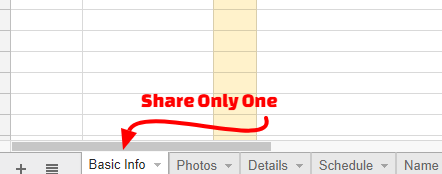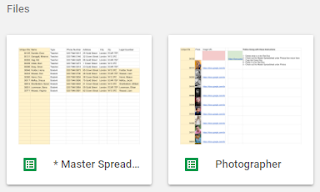The questions usually starts with "How do I Share a Single Tab in Google Sheets?" In this article I will cover what function to use and other useful formulas. (http://MrG.fyi/ShareTab)

There's a simple answer to that question. To share a single sheet or tab from a spreadsheet, you need to use the IMPORTRANGE function by creating multiple Google Sheets and then share.

## How?Fig. 1: One Spreadsheet Per Shared Tab
You will need a "Master Spreadsheet", with whatever name you want. For ease of explanation, mine is called "* Master Spreadsheet".
Next, you will need to create different Google Sheets that will have the data you want to share. Let's say I want to share photo data. I'll call the spreadsheet "Photographer" (see Figure 1 for example).
Now, use the IMPORTRANGE function on cell A1 as described on Figure 2.Fig. 2: Anatomy of the IMPORTRANGE function.

## Samples

When I learn something like this, it helps to have samples like the example above.
In the samples, I highlighted all the instances of the IMPORTRANGE. Plus, I have added a few more functions or formulas to help.

### FUNctions

#### =IMAGE("http://example.com/imageFile.jpg")

This allows images via URL, which can be stored in Google Drawings

#### Break a Comma Separated Name

For all these, I will pretend to have the name "Last, First" in cell A1. I want the First Name in B1 and Last Name in C1.
B1 =RIGHT(A1,LEN(A1)-FIND(",",A1)-1)
C1 =LEFT(A1,FIND(",",A1)-1)

#### If Then Else

An IF function has three parameters in this format IF(Condition, Then, Else). Or, If the condition is true Then do this, if the condition is false Else do this. For example
=IF(A1=B1, "GOOD JOB", "NICE TRY")

#### Display Content Based on Relative Location

Sometimes, I want to display something that is two columns right and 3 rows down from an unknown location. That location can be based on a search.

Example 1: You have a range, and you want to know what is available in a relative location where cell C2 has how deep and B2 how far. Index has three parameters (Range, Row, Column)
=INDEX('Data'!A:D,C2,B2)

Example 2: You have a search criteria for how deep, and you display the relative cell on that row. Vlookup has four parameters (Search Term, Range, Column, Is it Sorted). In this example C5 has the search term and D5 how far
=VLOOKUP(C5,'Data'!A:D,D5,FALSE)

## Using Google Drawings as Images

First: SHARE with who can see the image. For example, Anyone with the link can view.
Then, publish.

## Thank You!

Let me know what helped. What functions do you use?